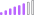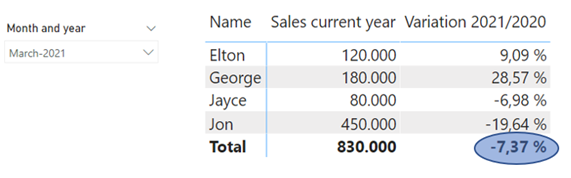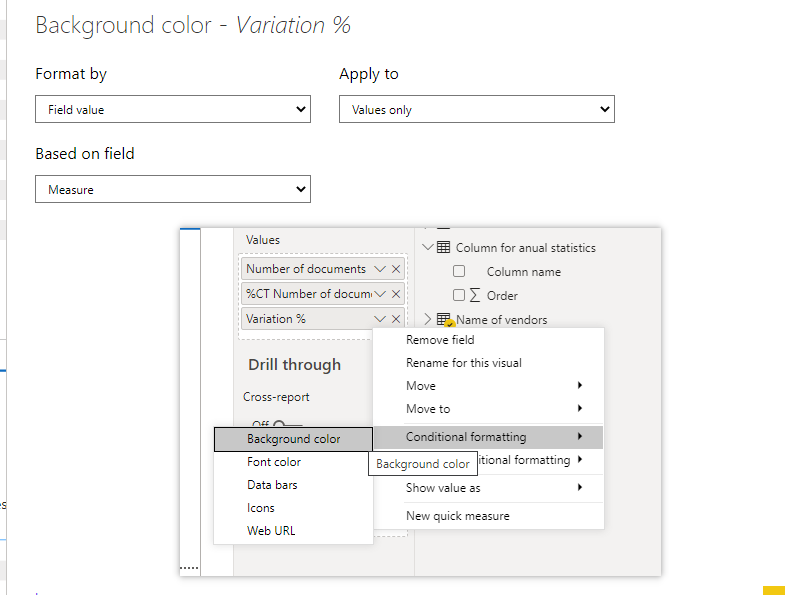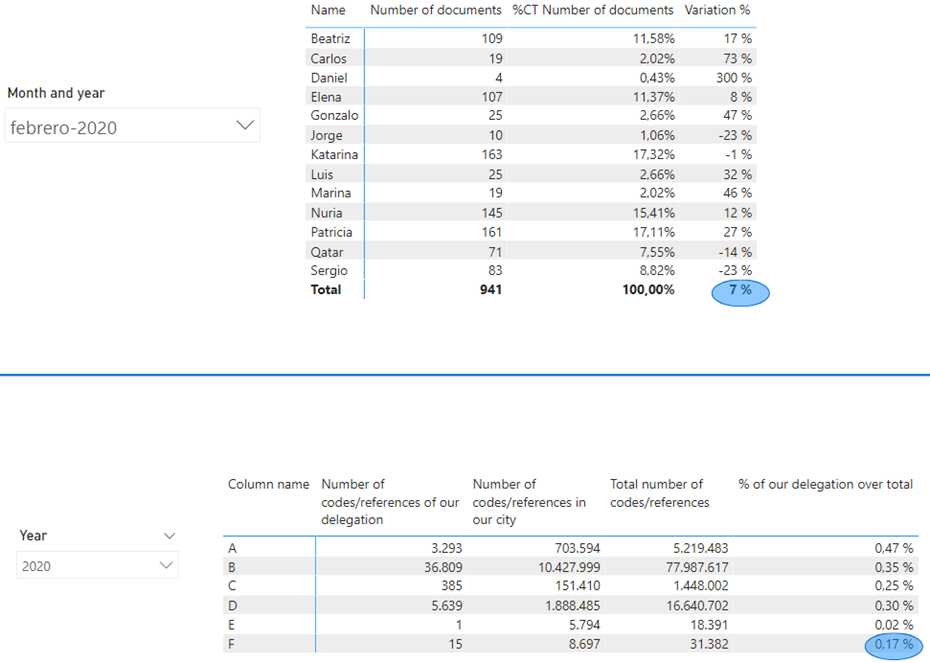cancel
Showing results for
Did you mean:Post Patron

## Get the total variation for applying a measure on it

Hi guys,

I want to get the variation of the last year to apply a measure on it, how could I get it?I have 3 tables:

Table 1. It is called Name and it contains the name of the sales agents.

Table 2. It is called Sales and it contains the sales transactions and the number of the sales agents.

Table 3. It is called Calendar table and it contains the calendar.

I have applied the measure:

CALCULATE(TOTALYTD(COUNTX(ALL(NAME);NAME[NAME]);CALENDAR TABLE [DATE]);NAME[MAIN OPERATION]="YES")

but it doesn't work.

Regards.
1 ACCEPTED SOLUTIONCommunity Support

Hi, @PwrBI01

The idea is that you need to calculate a single result separately.

Like this:

``````Measure =
VAR total =
SUMX (
SUMMARIZE (
ALL ( 'Name of vendors' ),
"a",
VAR CurrentPeriod = [Number of documents]
VAR PreviousPeriod =
CALCULATE (
[Number of documents],
DATEADD ( 'Calendar table'[Date], -12, MONTH )
)
RETURN
DIVIDE ( CurrentPeriod - PreviousPeriod, PreviousPeriod )
),
[a]
)
RETURN
SWITCH (
TRUE (),
[Variation %] > total, "green",
[Variation %] = total, "yellow",
"red"
)
``````The same is true for the other table, you need to calculate the value of F.

Best Regards

Janey Guo

If this post helps, then please consider Accept it as the solution to help the other members find it more quickly

9 REPLIES 9Post Patron

You are right, my explanation was really bad and it was really hard to understand it, so I will try to explain it in another way and bringing a data reference.

I have the following tables:And I want to create a conditional formatting that if the value is higher than the one with the blue circle it should be in green, if it is lower it should be in red and if it is similar it should be in yellow, as you can see in the following photo:I have created that for the first table but I also need the same for the second table, but it is similar.

I need to create a measure for obtain the value of the blue circles (one measure for each value) so then I can apply the following measure:

color measure =
var Total = -----------------------------------------
Return
switch(true();
[Variation %] > Total;"green";
[Variation %] = Total;"yellow";
[Variation %] < Total;"red")

What I need to create is the measure of the red part of the measure.

The file is in the following link: https://www.dropbox.com/s/604zd1onn39ox18/Problem%20conditional%20formatting%204.pbix?dl=0

Regards.Community Support

Hi, @PwrBI01

The idea is that you need to calculate a single result separately.

Like this:

``````Measure =
VAR total =
SUMX (
SUMMARIZE (
ALL ( 'Name of vendors' ),
"a",
VAR CurrentPeriod = [Number of documents]
VAR PreviousPeriod =
CALCULATE (
[Number of documents],
DATEADD ( 'Calendar table'[Date], -12, MONTH )
)
RETURN
DIVIDE ( CurrentPeriod - PreviousPeriod, PreviousPeriod )
),
[a]
)
RETURN
SWITCH (
TRUE (),
[Variation %] > total, "green",
[Variation %] = total, "yellow",
"red"
)
``````The same is true for the other table, you need to calculate the value of F.

Best Regards

Janey Guo

If this post helps, then please consider Accept it as the solution to help the other members find it more quicklyPost Patron

Thank you very much, it works correctly for the first table, but I don't know how to addapt it to use it for the second one.

Regards.Community Support

@PwrBI01  I have written it in the sample file, Please check it.

Best Regards

Janey Guo

If this post helps, then please consider Accept it as the solution to help the other members find it more quicklyPost Patron

I am really sorry, but I am trying to addapt it to a new table and I have a question for calculate 'del' inside 'Measure 2'.

Imagine we can sum A + B + C + D + E + F and we get the following total.

 Column Name Number of codes/references of our delegation del A 3.293 46.142 B 36.809 46.142 C 385 46.142 D 5.639 46.142 E 1 46.142 F 15 46.142 TOTAL 46.142 46.142

I want to get the right column called 'del' but when I take your measure:

``````VAR del =
CALCULATE (
SUM ( 'Reference table'[Number of references] );
FILTER (
ALL ( 'Column for anual statistics' );
'Column for anual statistics'[Column name] = "F"
);
FILTER ( 'Reference table'; 'Reference table'[Type number] = 99 );
USERELATIONSHIP ( 'Calendar table'[Date]; 'Reference table'[Date] )
)``````

And change it into the following one:

``````VAR del =
CALCULATE (
SUM ( 'Reference table'[Number of references] );
ALL ( 'Column for anual statistics' );
FILTER ( 'Reference table'; 'Reference table'[Type number] = 99 );
USERELATIONSHIP ( 'Calendar table'[Date]; 'Reference table'[Date] )
)``````

It doesn't work. There is a problem with all function (because it doesn't work, I can delete it and I get the same result) but I don't know how to correct it.

Regards.Community Support

@PwrBI01   The measure I wrote is for F. The conditions of abcdef in your measure are different, so of course you won’t get total.

Because your abcdef is different, total is not easy to calculate, you need to set the variable of abcdef in the measure, and then add them to get total.

If you can’t solve it, I suggest you open a new case and describe your problem.

Best Regards

Janey GuoPost Patron

@v-janeyg-msft I am trying to adapt it to a new table in which there is a same measure for abcdef. I just need to make that the measure takes the total and doesn't take into account a,b,c,d,e and f separately to use it, because the rest of the columns are similar to the second chart but in a same measure (they have a total for our delegation, total for all and I want to calculate our total over the global total).

Regards.Post Patron

Hi @v-janeyg-msft, thank you very much.Community Support

Hi, @PwrBI01

I really want to help you but your description doesn’t seem to be very clear, and there is no data reference.

Can you explain more details and share some sample data and your desired result like? So we can help you soon.

Best Regards

Janey guo